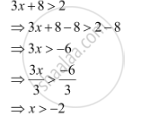CBSE (Arts) Class 11CBSE
Share

# Solve 3x + 8 > 2, When X is an Integer - CBSE (Arts) Class 11 - Mathematics

ConceptAlgebraic Solutions of Linear Inequalities in One Variable and Their Graphical Representation

#### Question

Solve 3x + 8 > 2, when  x is an integer

#### Solution

The given inequality is 3x + 8 > 2.The integers greater than –2 are –1, 0, 1, 2, …

Thus, when x is an integer, the solutions of the given inequality are

–1, 0, 1, 2 …

Hence, in this case, the solution set is {–1, 0, 1, 2, …}.

Is there an error in this question or solution?

#### APPEARS IN

NCERT Solution for Mathematics Textbook for Class 11 (2018 to Current)
Chapter 6: Linear Inequalities
Q: 4.1 | Page no. 122
Solution Solve 3x + 8 > 2, When X is an Integer Concept: Algebraic Solutions of Linear Inequalities in One Variable and Their Graphical Representation.
S